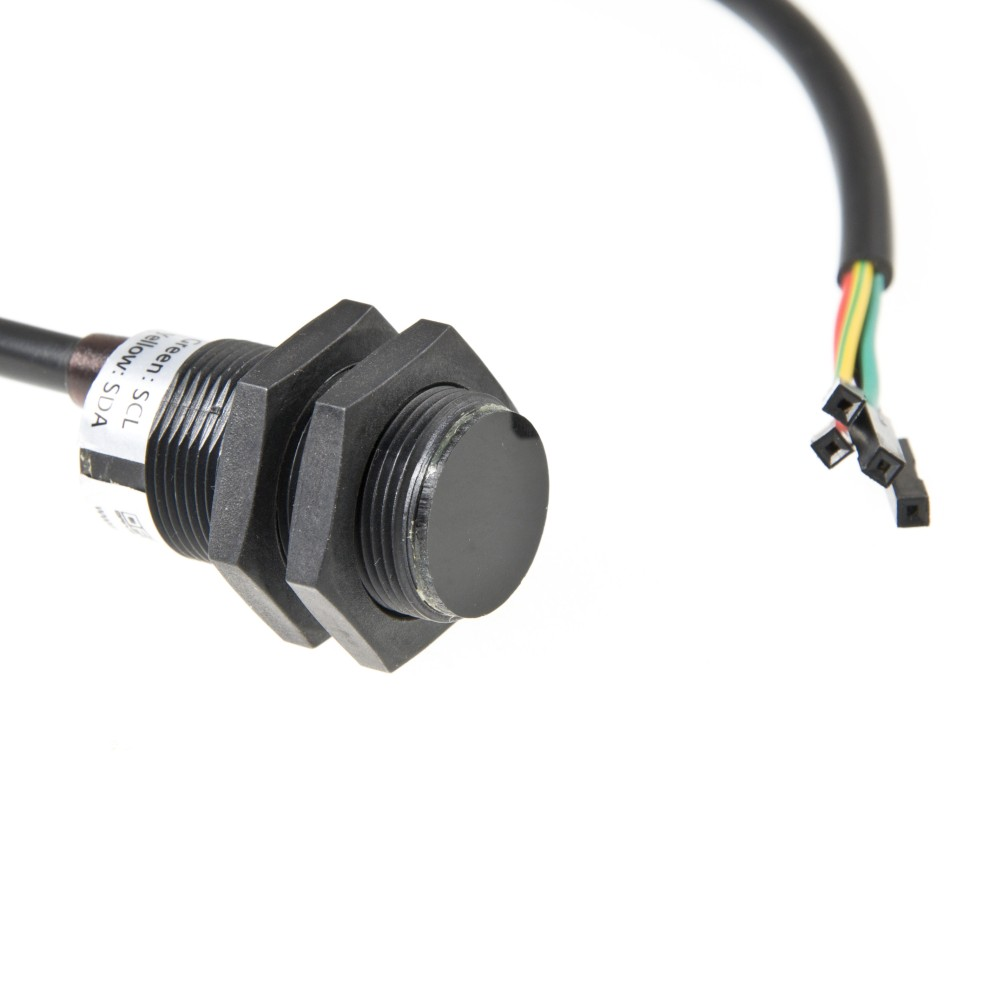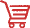# Positioning_ir_camera__SKU_SEN0158-DFRobot## Introduction

This camera is a small form positioning IR camera, which could track 4 IR objects at the same time. The applications are various and ranges from tracking robots with IR transmitters for navigation to light barriers, or determining an objects direction.

Additionally, it can also function as a flame sensor, which could track heat sources and a flames position.

The sensor is sasy to install and to connect. All you need is just four wires, two for power supply and two for I2C.

## Specification

• Power consumption requirements: 3.3V/44mA, 5V/48mA
• Detecting distance: 0~3m
• Communication protocol: I2C
• Horizontal detecting angle: 33 degrees
• Vertical detecting angel: 23 degrees
• Resolution is 128x96 pixel, with hardware image processing, which can track four objects (IR emitting or reflecting objects)
• Dimensions: 32 x 16 (cylinder shape)

• Red - VCC+
• Yellow - SDA
• Green - SCL
• Black - GND

## Connection Diagram## Sample Code

### Instruction

Once the camera detects a signal, it will display its coordinates on the first position. And others will be empty(returns 1023,1023). If the camera detects several objects, it will arrange them according to the detecting order. If one of them is out of the view, that position will be empty(return 1023,1023). It can only support tracking 4 objects at the same time.

### Arduino Code

``````// Wii Remote IR sensor  test sample code  by kako http://www.kako.com
// modified output for Wii-BlobTrack program by RobotFreak http://www.letsmakerobots.com/user/1433
// modified for https://dfrobot.com by Lumi, Jan. 2014

#include <Wire.h>

int ledPin = 13;
boolean ledState = false;
byte data_buf;
int i;

int Ix;
int Iy;
int s;

void Write_2bytes(byte d1, byte d2)
{
Wire.write(d1); Wire.write(d2);
Wire.endTransmission();
}

void setup()
{
slaveAddress = IRsensorAddress >> 1;   // This results in 0x21 as the address to pass to TWI
Serial.begin(19200);
pinMode(ledPin, OUTPUT);      // Set the LED pin as output
Wire.begin();
// IR sensor initialize
Write_2bytes(0x30,0x01); delay(10);
Write_2bytes(0x30,0x08); delay(10);
Write_2bytes(0x06,0x90); delay(10);
Write_2bytes(0x08,0xC0); delay(10);
Write_2bytes(0x1A,0x40); delay(10);
Write_2bytes(0x33,0x33); delay(10);
delay(100);
}
void loop()
{
ledState = !ledState;
if (ledState) { digitalWrite(ledPin,HIGH); } else { digitalWrite(ledPin,LOW); }

Wire.write(0x36);
Wire.endTransmission();

for (i=0;i<16;i++) { data_buf[i]=0; }
i=0;
while(Wire.available() && i < 16) {
i++;
}

Ix = data_buf;
Iy = data_buf;
s   = data_buf;
Ix += (s & 0x30) <<4;
Iy += (s & 0xC0) <<2;

Ix = data_buf;
Iy = data_buf;
s   = data_buf;
Ix += (s & 0x30) <<4;
Iy += (s & 0xC0) <<2;

Ix = data_buf;
Iy = data_buf;
s   = data_buf;
Ix += (s & 0x30) <<4;
Iy += (s & 0xC0) <<2;

Ix = data_buf;
Iy = data_buf;
s   = data_buf;
Ix += (s & 0x30) <<4;
Iy += (s & 0xC0) <<2;

for(i=0; i<4; i++)
{
if (Ix[i] < 1000)
Serial.print("");
if (Ix[i] < 100)
Serial.print("");
if (Ix[i] < 10)
Serial.print("");
Serial.print( int(Ix[i]) );
Serial.print(",");
if (Iy[i] < 1000)
Serial.print("");
if (Iy[i] < 100)
Serial.print("");
if (Iy[i] < 10)
Serial.print("");
Serial.print( int(Iy[i]) );
if (i<3)
Serial.print(",");
}
Serial.println("");
delay(15);
}``````

### Processing Code

``````// Example by Tom Igoe
// Modified for https://www.dfrobot.com by Lumi, Jan. 2014

/*
This code should show one colored blob for each detected IR source (max four) at the relative position to the camera.
*/

import processing.serial.*;

int lf = 10;    // Linefeed in ASCII
String myString = null;
Serial myPort;  // The serial port

void setup() {
// List all the available serial ports
println(Serial.list());
// Open the port you are using at the rate you want:
myPort = new Serial(this, Serial.list(), 19200);
myPort.clear();
// in the middle of a string from the sender.
myString = null;
size(800,800);
//frameRate(30);
}

void draw() {
background(77);
//while (myPort.available() > 0) {
if (myString != null) {
int[] output = int (split(myString, ','));

println(myString); // display the incoming string

int xx = output;
int yy = output;

int ww = output;
int zz = output;

int xxx = output;
int yyy = output;

int www = output;
int zzz = output;

fill(255, 0, 0);  // Set fill to white
ellipse(xx, yy, 20, 20);
fill(0, 255, 0);  // Set fill to white
ellipse(ww, zz, 20, 20);

fill(0, 0, 255);  // Set fill to white
ellipse(xxx, yyy, 20, 20);
fill(255);  // Set fill to white
ellipse(www, zzz, 20, 20);

}
}``````

## FAQ

Q&A Some general Arduino Problems/FAQ/Tips
Q What is the thread size on the SEN0158 part?
A It is M18.
Q In the manual, it mentions that the horizontal detecting angle is 33 degrees, while the vertical detecting angle is 23 degrees. How do I differentiate which is horizontal and which is vertical?Get Positioning ir camera from DFRobot Store or DFRobot Distributor.